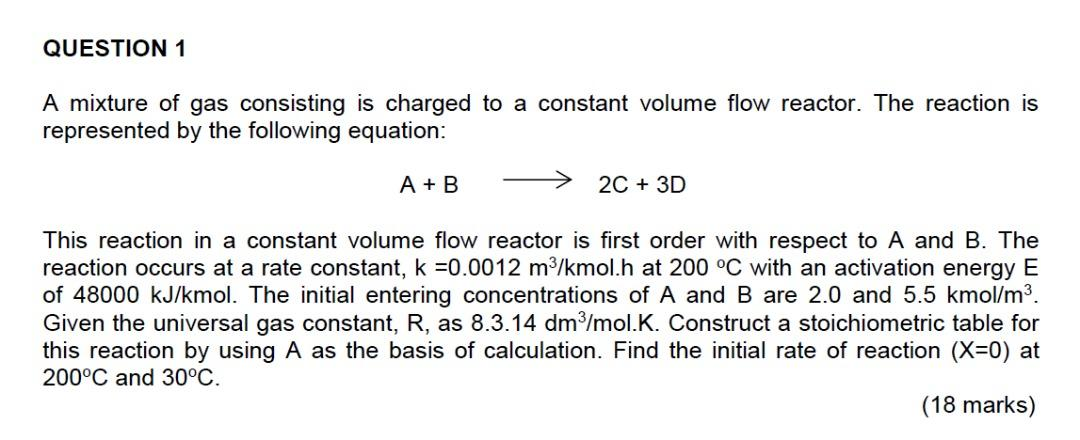# A mixture of gas consisting is charged to a constant volume flow reactor. The reaction is represented by the following equation: A + B 2C + 3D This reaction in a constant volume flow reactor is first order with respect to A and B. The reaction occurs at a rate constant, k =0.0012 m3/kmol.h at 200 °C with an activation energy E of 48000 kJ/kmol. The initial entering concentrations of A and B are 2.0 and 5.5 kmol/mº. Given the universal gas constant, R, as 8.3.14 dm3/mol.K. Construct a stoichiometric table for this reaction by using A as the basis of calculation. Find the initial rate of reaction (X=0) at 200°C and 30°C.

Question-AnswerCategory: Chemical Reaction EngineeringA mixture of gas consisting is charged to a constant volume flow reactor. The reaction is represented by the following equation: A + B 2C + 3D This reaction in a constant volume flow reactor is first order with respect to A and B. The reaction occurs at a rate constant, k =0.0012 m3/kmol.h at 200 °C with an activation energy E of 48000 kJ/kmol. The initial entering concentrations of A and B are 2.0 and 5.5 kmol/mº. Given the universal gas constant, R, as 8.3.14 dm3/mol.K. Construct a stoichiometric table for this reaction by using A as the basis of calculation. Find the initial rate of reaction (X=0) at 200°C and 30°C.A mixture of gas consisting is charged to a constant volume flow reactor. The reaction is represented by the following equation: A + B 2C + 3D This reaction in a constant volume flow reactor is first order with respect to A and B. The reaction occurs at a rate constant, k =0.0012 m3/kmol.h at 200 °C with an activation energy E of 48000 kJ/kmol. The initial entering concentrations of A and B are 2.0 and 5.5 kmol/mº. Given the universal gas constant, R, as 8.3.14 dm3/mol.K. Construct a stoichiometric table for this reaction by using A as the basis of calculation. Find the initial rate of reaction (X=0) at 200°C and 30°C.